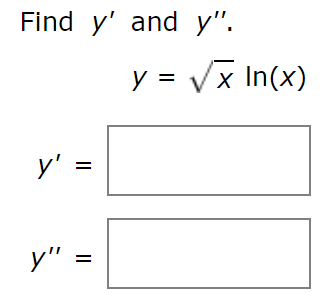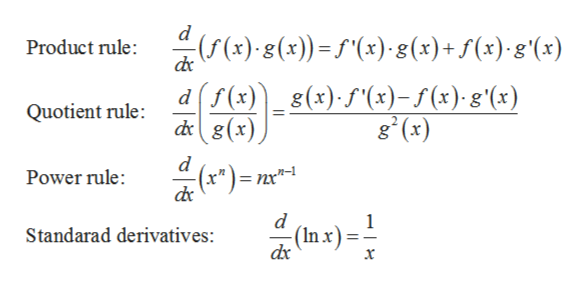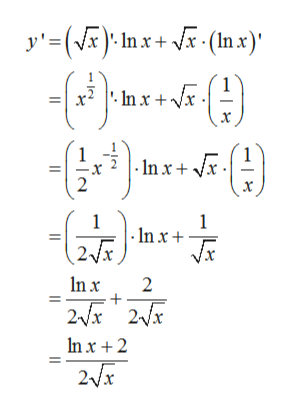Find y' and y".y x In(x)у"y'"

Questionhelp_outlineImage TranscriptioncloseFind y' and y". y x In(x) у" y'" fullscreen
Step 1

To find the first and second derivatives of the given function

Step 2

Since the function is a product of two functions, so we’ll use the following rules of derivatives:help_outlineImage Transcriptionclose(x)(x))x) 8(x)+ f(x)g(x) Product rule: f(x)g (x)f(x)-s (x). g'(x) dg(x) d Quotient rule: g (x) d n-1 Power rule: =nx"- d (Inx) Standarad derivatives: x fullscreen
Step 3

To find the first derivative, using the product ru...help_outlineImage Transcriptionclosey'=() Inx(x) 1 1 In x 2 1 |-In x 1 In x 2 2x2x In x 2 2x fullscreen

Want to see the full answer?

See Solution

Want to see this answer and more?

Our solutions are written by experts, many with advanced degrees, and available 24/7

See Solution
Tagged in

Calculus